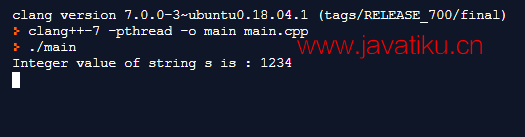# C++教程-C++转换为字符串## C++转换为字符串

C++中将整数转换为字符串有三种方法：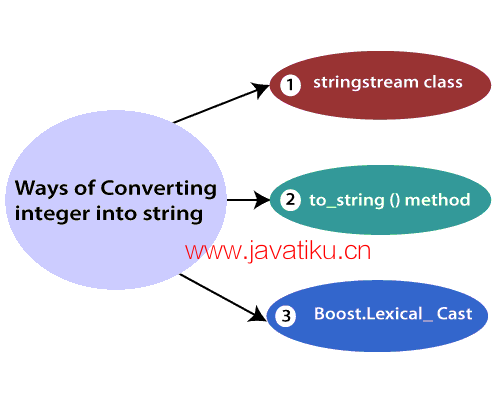• 使用stringstream类
• 使用to_string()方法
• 使用boost.lexical_cast

### 使用stringstream类将整数转换为字符串。

stringstream类是在头文件中定义的流类。它是用于在基于字符串的流上执行输入输出操作的流类。

• 运算符 >>：从流中提取数据。
• 运算符 <<：将数据插入流中。

• 在下面的语句中，<<插入运算符将100插入流中。

stream1 << 100;

• 在下面的语句中，>>提取运算符从流中提取数据，并将其存储在变量'i'中。

stream1 >> i;

``````#include <iostream>
#include <sstream>
using namespace std;
int main() {
int k;
cout << "Enter an integer value: ";
cin >> k;
stringstream ss;
ss << k;
string s;
ss >> s;
cout << "\n" << "An integer value is: " << k << "\n";
cout << "String representation of an integer value is: " << s;
}``````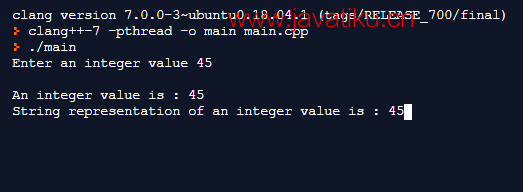``````cppCopy code
#include <iostream>
#include <sstream>
using namespace std;
int main() {
string number = "100";
stringstream ss;
ss << number;
int i;
ss >> i;
cout << "The value of the string is: " << number << "\n";
cout << "Integer value of the string is: " << i;
}``````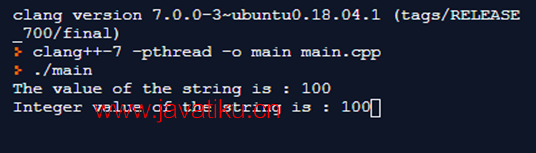### 使用to_string()方法将整数转换为字符串。

to_string()方法接受一个整数，并将整数值或其他数据类型值转换为字符串。

``````#include <iostream>
#include <string>
using namespace std;
int main() {
int i = 11;
float f = 12.3;
string str = to_string(i);
string str1 = to_string(f);
cout << "String value of integer i is: " << str << "\n";
cout << "String value of f is: " << str1;
}``````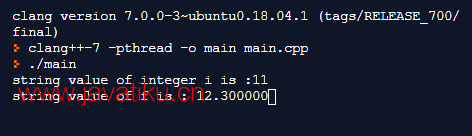### C++整数转字符串： 通过使用to_string函数将整数转换为字符串。

to_string函数接受一个整数作为参数，并返回一个表示该整数的字符串。

``````#include <iostream>
#include <boost/lexical_cast.hpp>
using namespace std;
int main()
{
int i = 11;
string str = boost::lexical_cast<string>(i);
cout << "整数i的字符串值为：" << str << "\n";
}``````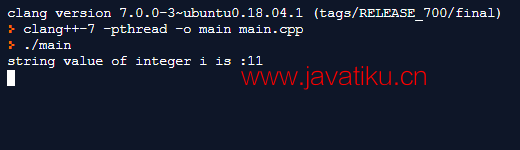C++字符串转整数： 通过使用boost::lexical_cast函数将字符串转换为整数。 boost::lexical_cast函数提供了一个转换运算符，即boost::lexical_cast，它可以将字符串值转换为整数值或其他数据类型值，反之亦然。

``````#include <iostream>
#include <boost/lexical_cast.hpp>
using namespace std;
int main()
{
string s = "1234";
int k = boost::lexical_cast<int>(s);
cout << "字符串s的整数值为：" << k << "\n";
}``````